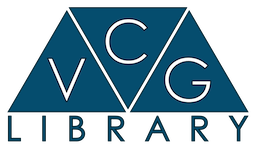VCG Library
vcg::vertex::Curvature< A, TT > Class Template Reference

Component: Per vertex basic curvature This component keeps the mean an gaussian curvature for a vertex. Used by some of the algorithms of vcg::tri::UpdateCurvature to store the computed curvatures. More...

`#include <component.h>`

Inherits TT.

## Detailed Description

### template<class A, class TT> class vcg::vertex::Curvature< A, TT >

Component: Per vertex basic curvature This component keeps the mean an gaussian curvature for a vertex. Used by some of the algorithms of vcg::tri::UpdateCurvature to store the computed curvatures.

Definition at line 414 of file vertex/component.h.

The documentation for this class was generated from the following file: